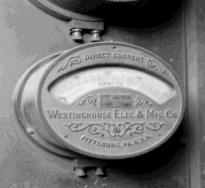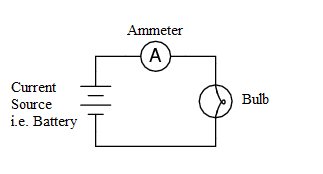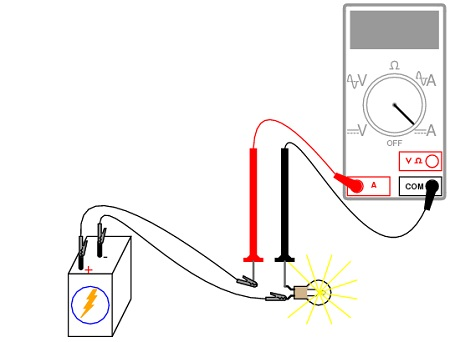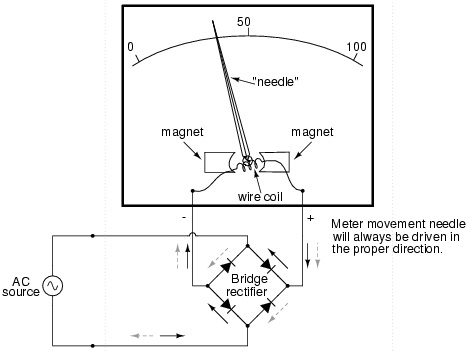# Ammeter Function Symbol & Definition

What is an ammeter? Ammeter or ampere meter is an electrical measuring device, which as seen from its nomenclature is useful for the measurement of the ampere i.e. to measure electric current through the circuit.

The normal symbol of ampere meter is capital A mentioned inside a circle. This is the way ammeter is symbolized in electric circuits.

History of ammeter:

Hans Christian Orsted in 1820 discovered the relation between electric current, magnetic fields and physical forces. His observations revealed that a compass needle deflects from pointing North when a current flows in an adjacent wire. Below is the image of first ampere meter:

##Ammeter Function & Usage

The usage of ampere meter is to measure the flow of electrical current in a circuit. As a principle any measuring devices should not affect the circuit which is under the study, therefore for ammeter, it is important that the internal resistance is very small (ideally near zero) so that the measuring device is not disturbing the actual circuit i.e. the flow of current is not disturbed.This is very important that how is an ammeter connected to a circuit, because if the ammeter is connected across a voltage difference, it will conduct a large current and ammeters will become faulty. Therefore, whenever you want to understand that how to connect ammeter,  remember that it must be connected in series in a circuit, never in parallel with a voltage source.

In addition to internal damage high currents may also damage the needle on an analog ammeter. The high current cause the needle to move too quickly, hitting the pin at the end of the scale. Always set the ammeter to the highest scale possible, then adjust downward to the appropriate level.### Types of ammeter / ampere meter

There are different types of ampere meters as mentioned below:

• Moving-coil ammeters
• Electrodynamic ammeters
• Moving-iron ammeters
• Hot-wire ammeters
• Digital ammeters
• Integrating ammeters

Moving-coil ammeter uses magnetic deflection, where current passing through a coil causes the coil to move in a magnetic field.

Electrodynamic ammeter uses an electromagnet instead of the permanent magnet of the d’Arsonval movement.

Moving-iron ammeter uses a piece of iron which moves when acted upon by the electromagnetic force of a fixed coil of wire. In a hot-wire ammeter, a current passes through a wire which expands as it heats.

Digital Ammeter uses a shunt resistor to produce a calibrated voltage proportional to the current flowing.

For integrating ammeter the current is summed over time, giving as a result the product of current and time; which is proportional to the energy transferred with that current.

#### Ammeter vs Voltmeter

If we compare ammeter vs voltmeter the difference is very clear that ampere meter measures the flow of current in the form of Amperes and voltmeter measures the potential difference in volts.

Voltmeters are used to measure the potential difference between two points. Since the voltmeter should not affect the circuit, the voltmeters have very high (ideally infinite) impedance. Thus, the voltmeter should not draw any current, and not affect the circuit. In general, all devices have physical limits. These limits are specified by the device manufacturer and are referred to as the device rating. The ratings are usually expressed in terms of voltage limits, current limits, or power limits. It is up to the operating engineer to make sure that in device operation, these ratings (limit values) are not exceeded.

error: Content is protected !!